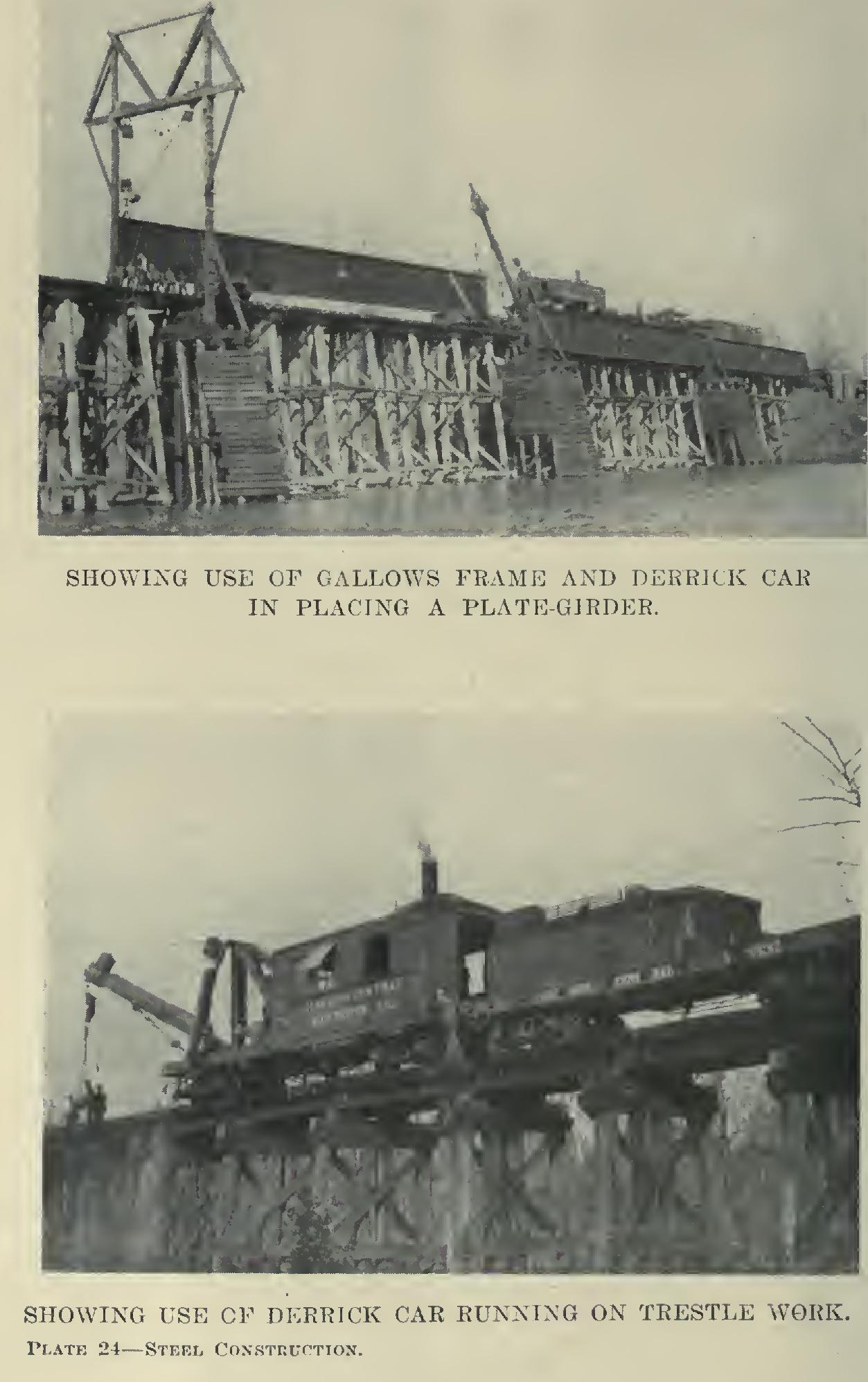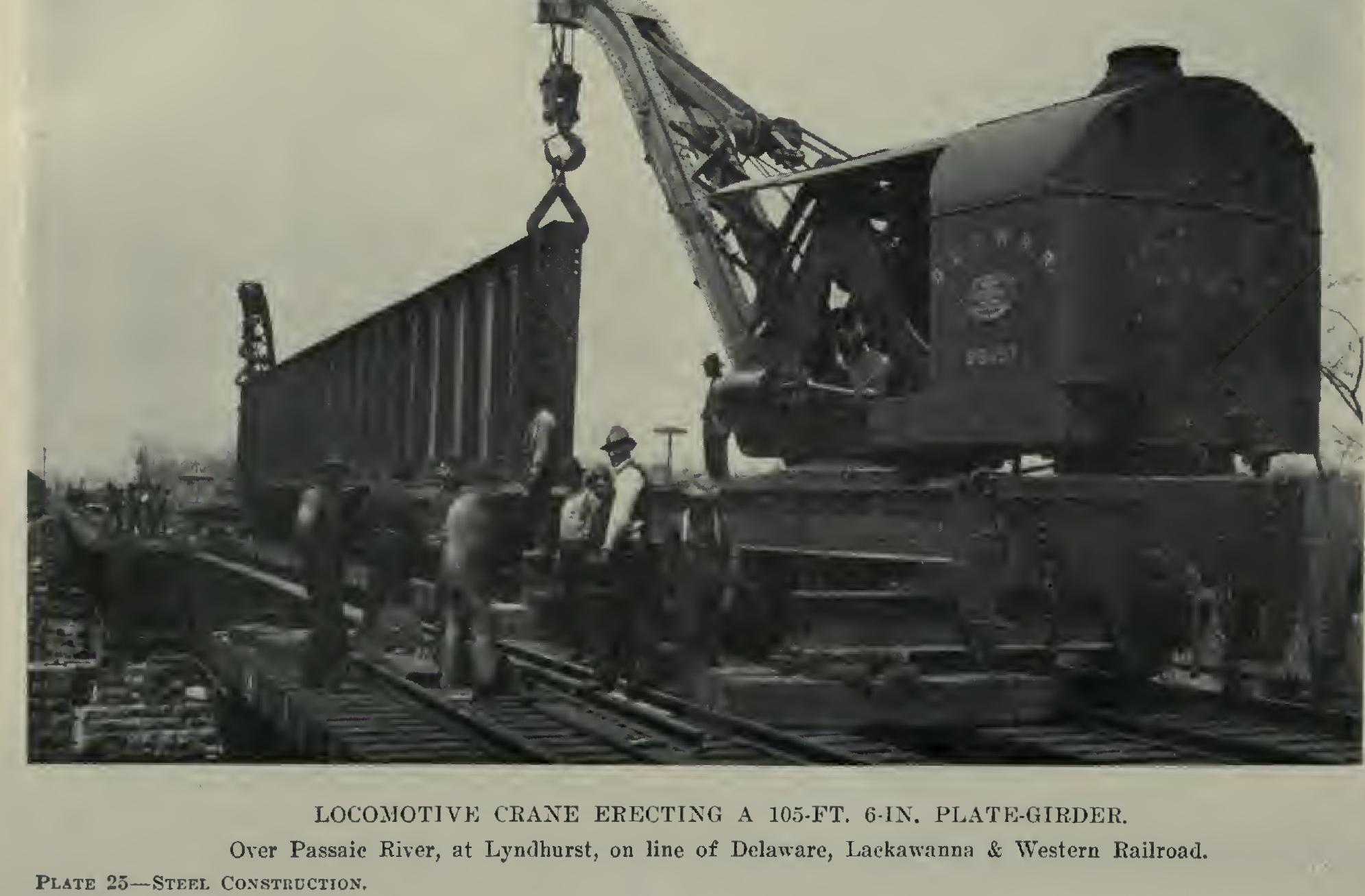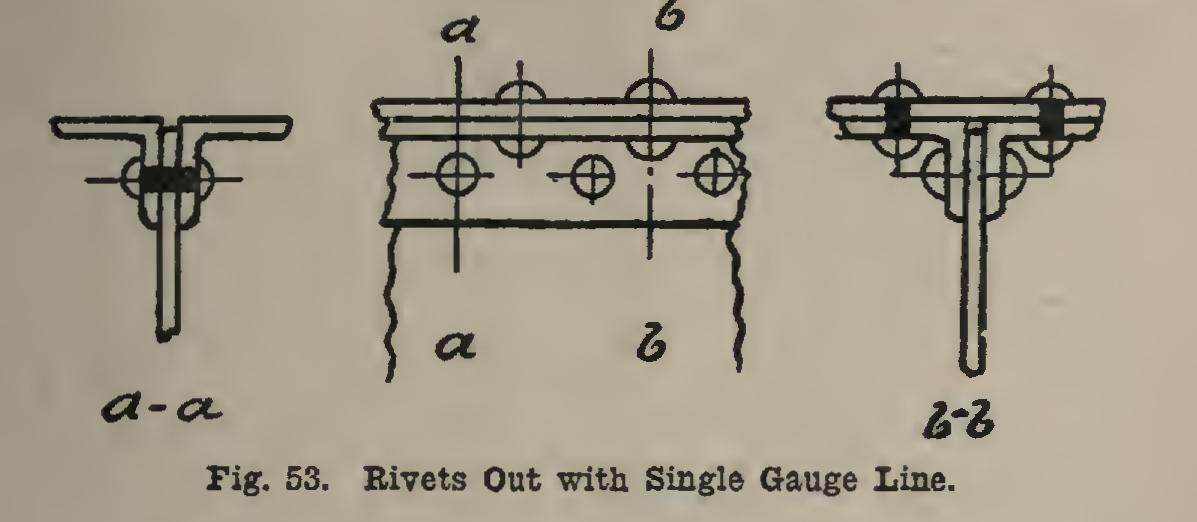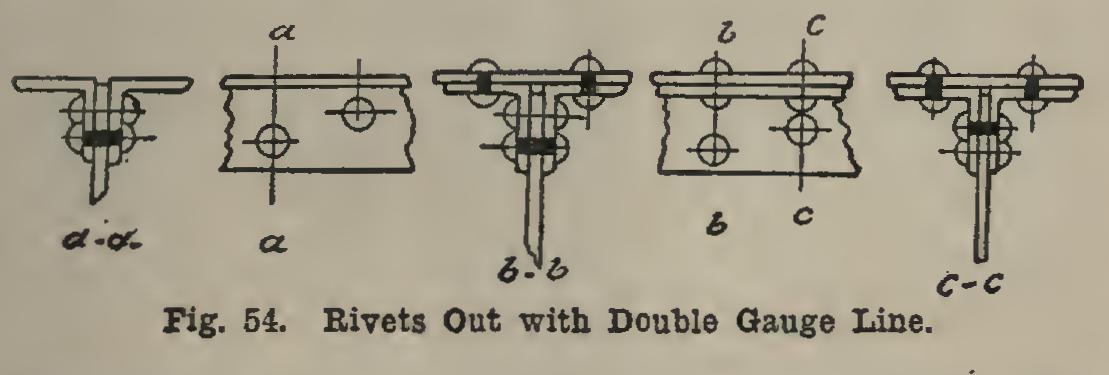# Girders 40

## angles, inch, cover-plate, table, angle, plate and value

Page: 1 2 3 4 5 6 7

The design of plate-girders for unit allow able stresses other than 15,000 pounds per square inch, and for either uniform or concen trated loads, is done by use of the maximum moment which the loads produce.

Thicknesses of flange angles greater than 13/16 inch should be avoided, since it will be necessary to bore the holes instead of punching them, and this increases the cost.

Angles should have the large leg turned out if they are unequal-legged, the small leg being riveted to the web plate.

Web plates should never be thinner than % inch, since they would not be stiff enough if they were.

The design of plate-girders may be made by the help of Tables XI and XII.

Rules— (1) Having the moment due to the loads given, assume a given angle; divide it by the height less the value x, column 4, if a cover-plate is not to be used; and then divide the result by the allowable unit-stress. The result will be a, column 3, and the required angles can be picked out of column 1, Table XL (2) Compute the thickness of the web plate; and if this comes out thinner than inch, use e- inch.

(3) Compute the weight of the girder as now de signed.

(4) Compute the bending moment due to its own weight.

(5) Add the bending moment due to its own weight to that due to the loads, and repeat operation (1). The result will be the section of the girder.

If it is desired to have a cover-plate on the girder, assume the sized angle as before, and, using y and b in place of x and a, proceed as above.

Cover-plates less than 7/16 inch run the en tire length of the girder. If the total thickness of a cover-plate runs up to inch, it should be composed of two plates, one inch thick, and the other inch or less. If the cover-plate runs up to inches, there should be three cover-plates, each inch thick. In all cases the thinnest plate should be on the outside of the girder. If there are more cover-plates than one, the length may be determined from Table XII.

Rules— (1) Compute the area of the cover-plates from the top to the under side of the one whose length is desired. This is readily done by multiplying the value in column 8 of Table XI by the number of sixteenths the plate is thick.

(2) Divide the area of the cover-plate so computed by the value b., column 6, Table XI, if there be one 1 inch only. If the plate is thicker than inch, then divide the area of the cover-plate as computed above, by the value given in column 6, after the additional amount as per column 8 has been added to it.

(3) In Table XII find the ratio A which is equal to the value just obtained, or next above it in value, and just below will be found the coefficient Q.

(4) Multiply the span of the girder by Q, and the result will be the length of the cover-plate on the bottom.

One thing should be avoided. No section should be used for a flange in which b, column 6, Table XI, is over twice as great as the value a, but it shows good designing to have it almost twice as great as a.

Angles marked with a * in Table XI have one rivet-hole out of the section of each angle; and when a cover-plate is used, two rivet-holes are out of this also (see Fig.

53). Angles not marked have one rivet-hole taken out of each angle; and when a cover-plate is used, two rivet-holes are taken out of each angle, and two out of the cover-plate (see Fig.

## 54). It

will be noticed in Figs. 53 and 54, that the web plate does not extend up to the back of the angles. This is done in order that, if any little irregularities occur in the rolling, they will not stick out above the backs of the angle. This distance is usually made inch in practice, and Table XI is figured on that basis. From Fig. 53 it is seen that the rivets in the cover-plates must not come opposite those in the leg of the angle which lies against the web, while in the angle of Fig. 54 this may be done.In Table XI the value a is the area of the two angles after the two rivet-holes have been subtracted; and b is the area of the cover-plate and angles after rivet-holes have been taken out as per Figs. 53 and 54.The values a and y are the distances from the edge of the web plate to the center of gravity axis of the angle or angles and plates. These values vary slightly with the thickness of the angles, and also with the thickness of the cover plate; but for practical purposes are constant as shown. The center of gravity of a pair of angles, or of two angles and a cover-plate, is that point at which, if they were supported, they would just balance (see Fig. 55). And the cen ter of gravity axis is a line passing through this point and parallel to the other leg of the angles, as shown in Fig. 55.Page: 1 2 3 4 5 6 7